# The GLMSELECT Procedure

#### Elastic Net Selection (ELASTICNET)

The elastic net method bridges the LASSO method and ridge regression. It balances having a parsimonious model with borrowing strength from correlated regressors, by solving the least squares regression problem with constraints on both the sum of the absolute coefficients and the sum of the squared coefficients. More specifically, the elastic net coefficients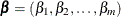are the solution to the constrained optimization problem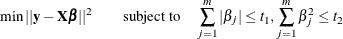The method can be written as the equivalent Lagrangian form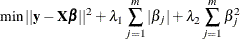If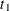is set to a very large value or, equivalently, if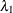is set to 0, then the elastic net method reduces to ridge regression. If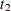is set to a very large value or, equivalently, if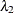is set to 0, then the elastic net method reduces to LASSO. Ifandare both large or, equivalently, ifandare both set to 0, then the elastic net method reduces to ordinary least squares regression.

As stated by Zou and Hastie (2005), the elastic net method can overcome the limitations of LASSO in the following three scenarios:

• In the case where you have more parameters than observations,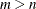, the LASSO method selects at most n variables before it saturates, because of the nature of the convex optimization problem. This can be a defect for a variable selection method. By contrast, the elastic net method can select more than n variables in this case because of the ridge regression regularization.

• If there is a group of variables that have high pairwise correlations, then whereas LASSO tends to select only one variable from that group, the elastic net method can select more than one variable.

• In the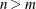case, if there are high correlations between predictors, it has been empirically observed that the prediction performance of LASSO is dominated by ridge regression. In this case, the elastic net method can achieve better prediction performance by using ridge regression regularization.

An elastic net fit is achieved by building on LASSO estimation, in the following sense. Let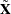be a matrix obtained by augmentingwith a scaled identity matrix,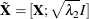Let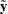be a vector correspondingly obtained by augmenting the responsewith m 0’s,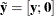Then the Lagrangian form of the elastic net optimization problem can be reformulated as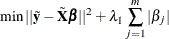In other words, you can solve the elastic net method in the same way as LASSO by using this augmented design matrixand response. Therefore, for given, the coefficients of the elastic net fit follow the same piecewise linear path as LASSO. Zou and Hastie (2005) suggest rescaling the coefficients by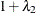to deal with the double amount of shrinkage in the elastic net fit, and such rescaling is applied when you specify the ENSCALE option in the MODEL statement.

If you have a good estimate of, you can specify the value in the L2= option. If you do not specify a value for, then by default PROC GLMSELECT searches for a value between 0 and 1 that is optimal according to the current CHOOSE= criterion. Figure 48.12 illustrates the estimation of the ridge regression parameter(L2). Meanwhile, if you do not specify the CHOOSE= option, then the model at the final step in the selection process is selected for each(L2), and the criterion value shown in Figure 48.12 is the one at the final step that corresponds to the specified STOP= option (STOP=SBC by default).

Figure 48.12: Estimation of the Ridge Regression Parameter(L2) in the Elastic Net Method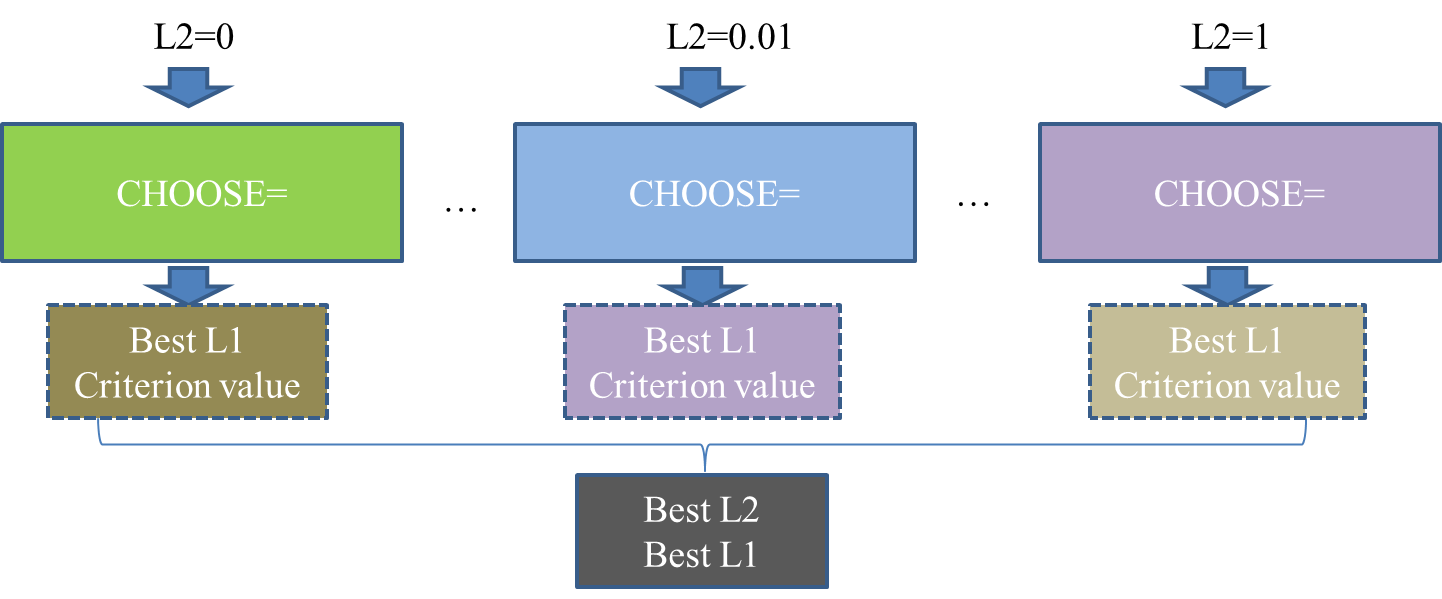Note that when you specify the L2SEARCH=GOLDEN, it is assumed that the criterion curve that corresponds to the CHOOSE= option with respect tois a smooth and bowl-shaped curve. However, this assumption is not checked and validated. Hence, the default value for the L2SEARCH= option is set to GRID.